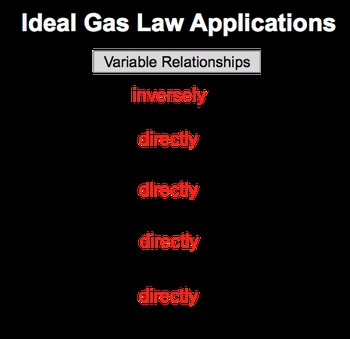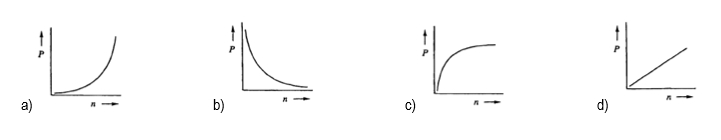## General Chemistry

Learn the toughest concepts covered in Chemistry with step-by-step video tutorials and practice problems by world-class tutors

7. Gases

# The Ideal Gas Law Applications

The Ideal Gas Law Applications establish both the direct and inverse relationships between the Ideal Gas Law variables.

Understanding the Ideal Gas Law Applications
1
concept

## The Ideal Gas Law Applications4m
Play a video:
By rearranging the ideal gas law, we can establish direct and inverse relationships between its variables. So here we have the ideal gas law formula, which is PV equals NRT. Our is our gas constant because it's constant. We don't have to relate it to the other variables. Next we have our variable chart, and we'll see what effect happens to the other variables. If pressure is increasing and volume is increasing, and then in our variable relationships will take a look at different pairs of variables and see, Are they directly proportional or inversely proportional to one another? All right, so first, let's look at what happens when I increased pressure. Let's see, what is the effect that it has on volume? Our equation is PV equals NRT. We're not gonna talk about NRT because we're looking strictly at the relationship between pressure and volume. Now to make them relate to one another, we're going to say that this is equal to a constant. Let's just say that it's one and we're gonna bring volume over to the other side. By doing that, we can see that the relationship is that pressure is equal to one over V pressure here is a numerator. Volume is a denominator. If you've watched my other videos in terms of direct and inverse relationships, realized that because they're on different levels, they're inversely proportional. If volume is increasing and the top is staying, constant volume is increasing. That means pressure would be decreasing. If volume is decreasing, pressure would be increasing. So coming back to our variables chart, we're going to say that the relationship is if we increase my pressure, that's going to decrease my volume because they have an inversely proportional relationship or opposite relationship. Now let's compare pressure and moles. Okay, so pressure and moles ignore volume are anti. So then this would just be pressure equals moles. They're both enumerators, both on the same level. If I increase one, that's gonna cause an increase in the other. So increasing pressure, while keeping all the other variables out, increases my moles. Since they're increasing or decreasing together, they are directly proportional. Next pressure equals temperature, right? Ignore volume moles and are because we're focusing on Leon pressure and temperature again. They're both numerator, so they both increased together. So they are directly proportional. All right, now that we've done that Let's look at volume. So volume were increased in volume and we're trying to see what effect it has on my moles and my temperature here. So focus on Onley, Moles and volume Ignore pressure, Ignore our anti volume and moles are on the same level with one another. They're both numerator, so increasing one would cause an increase in the other. Why? Because they are directly proportional and then finally volume equals temperature. So at this point you've seen us. Do the other ones give yourself a second pause the video if you want and see what the relationship between volume and temperature would be, all right. Hopefully you've done that and realize that volume and temperature, both of them considered numerator is both on the same level. An increase in one would cause an increase in the other. Why? Because they too would be directly proportional. So these are the relationships that we can establish between the variables of the ideal gas law. And we can see that really only pressure and volume is where we would see an inverse relationship when doing these pairings of the variables. So keep this in mind if you're faced with any type of theoretical question where they're increasing or decreasing one variable and asking for the effect on another within the ideal gas law.2
example

## The Ideal Gas Law Applications Example 11m
Play a video: